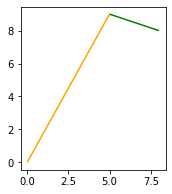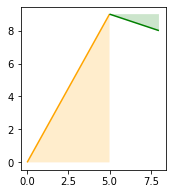# Introduction

I am writing this post just to cement in my mind how to calculate a turn angle from one OSM segment to another. This is pretty basic geomertry but I tripped myself up last week and made a simple mistake that left me confused for way too long, as is tradition. At any rate, hoping to write this down both for myself and in case anyone else needs a dumbed down explanation of how to calculate if something is a right hand turn or a left hand turn and how much it is one or the other (a sharp right? a sharp left? for example).

# Simple example set up

First we can create 2 example segments. Both will just have 2 coordinates (start node and end node). One leads to the next (so one way in OSM leading to another at an intersection). I will represent these as LineString objects and we can view them with GeoPandas:

This will render the following:## Calculating angles

First, we can determine the angle for each with a simple arctan calculation. We do this by generating a right triangle (as shown) for each and calculating the inverse of tangent (opposite over adjacent).

Now we can see the two triangles as determines using the x and y offsets:We can now calculate the angle for each triangle with the following:

This prints the following:

We can also go ahead and normalize each of these to non-negative angles and consider this the bearing of each angle as a value between 0 and 360:

The values for each will be: